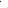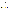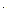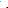# The Relationship Between Average and Marginal Costs

## Average cost drops in quantity produced when marginal cost is below average costAndy Baker / Getty Images

There are several ways to measure the costs of production, and some of these costs are related in interesting ways.  For example, average cost (AC), also called average total cost, is the total cost divided by quantity produced; marginal cost (MC) is the incremental cost of the last unit produced. Here's how  average cost and marginal cost are related:

## Analogy for Average and Marginal Cost RelationshipJodi Beggs

The relationship between average and marginal cost can be easily explained via a simple analogy. Rather than think about costs, think about grades on a series of exams.

Assume that your average grade in a course is 85. If you were to get a score of 80 on your next exam, this score would pull your average down, and your new average score would be something less than 85. Put another way, your average score would decrease.

If you scored 90 on that next exam, this grade would pull your average up, and your new average would be something greater than 85. Put another way, your average score would increase.

If you scored 85 on the exam, your average would not change.

Returning to the context of production costs, think of average cost for a particular production quantity as the current average grade and marginal cost at that quantity as the grade on the next exam.

One typically thinks of marginal cost at a given quantity as the incremental cost associated with the last unit produced, but marginal cost at a given quantity can also be interpreted as the incremental cost of the next unit. This distinction becomes irrelevant when calculating marginal cost using very small changes in quantity produced.

Following the grade analogy, average cost will be decreasing in quantity produced when marginal cost is less than average cost and  increasing in quantity when marginal cost is greater than average cost. Average cost will be neither decreasing nor increasing when marginal cost at a given quantity is equal to average cost at that quantity.

## Shape of Marginal Cost CurveJodi Beggs

The production processes of most businesses eventually result in diminishing marginal product of labor and diminishing marginal product of capital, which means that most businesses reach a point of production where each additional unit of labor or capital isn't as useful as the one that came before.

Once diminishing marginal products is reached, the marginal cost of producing each additional unit will be greater than the marginal cost of the previous unit. In other words, the marginal cost curve for most production processes will eventually slope upward, as shown here.

## Shape of Average Cost CurvesJodi Beggs

Because average cost includes fixed cost but marginal cost does not, it is generally the case that average cost is greater than marginal cost at small quantities of production.

This implies that average cost generally takes on a U-type shape, since average cost will be decreasing in quantity as long as marginal cost is less than average cost but then will start increasing in quantity when marginal cost becomes greater than average cost.

This relationship also implies that average cost and marginal cost intersect at the minimum of the average cost curve. This is because average cost and marginal cost come together when average cost has done all its decreasing but hasn't started increasing yet.

## Relationship Between Marginal and Average Variable CostsJodi Beggs

A similar relationship holds between marginal cost and average variable cost. When marginal cost is less than average variable cost, average variable cost is decreasing. When marginal cost is greater than average variable cost, average variable cost is increasing.

In some cases, this also means that average variable cost takes on a U-shape, though this is not guaranteed since neither average variable cost nor marginal cost contains a fixed cost component.

## Average Cost for Natural MonopolyJodi Beggs

Because marginal cost for a natural monopoly doesn't increase in quantity as it eventually does for most firms, average cost takes on a different trajectory for natural monopolies than for other firms.

Specifically, the fixed costs involved with a natural monopoly imply that average cost is greater than marginal cost for small quantities of production. The fact that marginal cost for a natural monopoly doesn't increase in quantity implies that average cost will be greater than marginal cost at all production quantities.

This means that, rather than being U-shaped, average cost for a natural monopoly is always declining in quantity, as shown here.

Format
mla apa chicago
Your Citation
Beggs, Jodi. "The Relationship Between Average and Marginal Costs." ThoughtCo, Feb. 16, 2021, thoughtco.com/relationship-between-average-and-marginal-cost-1147863. Beggs, Jodi. (2021, February 16). The Relationship Between Average and Marginal Costs. Retrieved from https://www.thoughtco.com/relationship-between-average-and-marginal-cost-1147863 Beggs, Jodi. "The Relationship Between Average and Marginal Costs." ThoughtCo. https://www.thoughtco.com/relationship-between-average-and-marginal-cost-1147863 (accessed June 24, 2021).